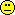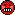wienui
★

Germany/Oman,
2022-04-15 09:44
(41 d 09:00 ago)

Posting: # 22932
Views: 791

## New FDA bioavailability guidance [BE/BA News]

Dear All,

Yesterday, on 14 April, The US Food and Drug Administration (FDA) published final guidance on the bioavailability (BA) data drug sponsors should include in their premarket investigational new drug (IND) applications, new drug applications (NDA), and NDA supplements.

The guidance has been several years in the making after garnering significant feedback from the industry, which the agency says it has addressed in the latest version.

Cheers,
Osama
Relaxation
★

Germany,
2022-04-16 13:59
(40 d 04:45 ago)

@ wienui
Posting: # 22933
Views: 572

## New FDA bioavailability guidance

Thanks.

Always great that the community finds time to let us know of such events.
Now I only have the task to find time to read through and compare to the draft and the European approach this week.

Happy Easter to all of you!

Relaxation
zizou
★

Plzeň, Czech Republic,
2022-04-16 18:22
(40 d 00:22 ago)

@ Relaxation
Posting: # 22934
Views: 544

## New FDA bioavailability guidance

Hello.

What suprised me is a change of rounding of CI in Appendix A (last point).

Draft:

1024 Rounding off of confidence interval values
1025
1026 We recommend that applicants not round off CI values; therefore, to pass a CI limit of 80 to 125
1027 percent, the value should be at least 80.00 percent and not more than 125.00 percent.

Final:

G. Confidence Interval Values for Unscaled Average Bioequivalence Analyses

Sponsors should round off CI values to two decimal places. For unscaled average bioequivalence analyses, to pass a confidence interval limit of 80 to 125 percent, the rounded confidence interval value should be at least 80.00 percent and not more than 125.00. We thus recommend that when applicants evaluate the confidence interval to assess bioequivalence using an unscaled average bioequivalence analysis during the development program, applicants round confidence interval values to two digits after the decimal point.

This change is equivalent to the change of acceptance interval from [80,125] to [79.995,125.005). So we can correct acceptance limits in sample size calculations (just to be exact) and also edit the procedure (coded in statistical software) with comparison of exact values of CI with acceptance limits (round CI before comparison or equivalently change the acceptance limits).
So it will be the same as according to EMA guideline now.

I was hoping for the change of EMA guideline to not round off CI values. Now, I would write the acceptance interval [79.995,125.005) in the study protocols as these values are exact and no questions about TIE > 5 % can arise! ;D

Best regards,
zizou
Helmut
★★★Vienna, Austria,
2022-04-17 13:03
(39 d 05:41 ago)

@ zizou
Posting: # 22935
Views: 533

## Excellent post!

Hi zizou,

congratulations for discovering this ‘goody’!

»

G. Confidence Interval Values for Unscaled Average Bioequivalence Analyses
»
» Sponsors should round off CI values to two decimal places. […]

» This change is equivalent to the change of acceptance interval from [80,125] to [79.995,125.005).

Yessir! Almost (see at then end).

» So we can correct acceptance limits in sample size calculations (just to be exact) and also edit the procedure (coded in statistical software) with comparison of exact values of CI with acceptance limits (round CI before comparison or equivalently change the acceptance limits).
» So it will be the same as according to EMA guideline now.Splendid.

» I was hoping for the change of EMA guideline to not round off CI values.

So did I. Everything else is bad science.
For my thinking about ‘nice numbers’ and rounding see there.

I think that the ‘experts’ of the FDA forgot (or worse: were not aware of) the origin of the limits, namely the clinically relevant difference $$\small{\Delta}$$:
$$\left\{\theta_1,\theta_2\right\}=\left\{100\,(1-\Delta),100/(1-\Delta)\right\}\tag{1}$$ We get ‘nice numbers’ of exactly $$\small{\left\{80\%,125\%\right\}}$$ only for $$\small{\Delta=20\%}$$. Since we analyze $$\small{\log_{e}}$$-transformed data, the limits are actually $$\small{\mp 0.22314355131421\ldots}$$ Not so nice.
Possibly the FDA is not aware of other jurisdictions where $$\small{\Delta=10\%}$$ is applicable for NTIDs or $$\small{\Delta=25\%}$$ for HVD(P)s. Then the upper limits are periodic ($$\small{\dot{1}11.11\%}$$ and $$\small{1\dot{3}3.33\%}$$).

» Now, I would write the acceptance interval [79.995,125.005) in the study protocols as these values are exact and no questions about TIE > 5 % can arise! ;DOh dear! See there. $$\small{125.005}$$ only if the software supports scientific rounding. Crappy software apply commercial rounding and then you would have to state $$\small{124.004\dot{9}}$$.

We can calculate the realized $$\small{\widehat\Delta_\textrm{r}}$$ from the limits: $$\widehat\Delta_\textrm{r}=\left\{\begin{array}{ll}\tag{2} 100\,(1-\theta_1/100)\\ 100\,(1-100/\theta_2) \end{array}\right.$$ Works as expected with the exact limits ($$\small{\widehat\Delta_\textrm{r}\equiv\Delta}$$) and we get what we desired. However with $$\small{79.995\%}$$ and $$\small{125.005\%}$$ we get $$\small{20.005\%}$$ and $$\small{20.0031\dot{9}\%}$$, which are larger than the $$\small{\Delta=20\%}$$ not only the FDA assumed. That’s the inflated Type I Error you wrote about. Not rocket science.Dif-tor heh smusma 🖖Helmut SchützThe quality of responses received is directly proportional to the quality of the question asked. 🚮
Science QuotesIng. Helmut Schütz